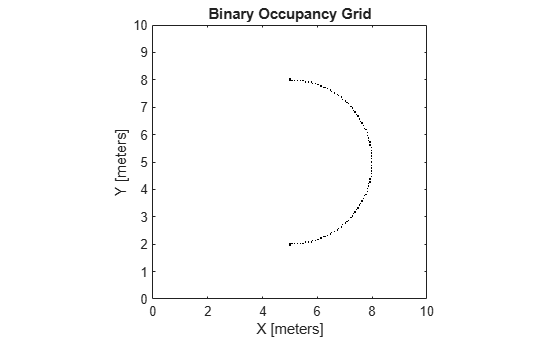# insertRay

Insert ray from laser scan observation

## Syntax

``insertRay(map,pose,scan,maxrange)``
``insertRay(map,pose,ranges,angles,maxrange)``
``insertRay(map,startpt,endpoints)``

## Description

example

````insertRay(map,pose,scan,maxrange)` inserts one or more lidar scan sensor observations in the occupancy grid, `map`, using the input `lidarScan` (Robotics System Toolbox) object, `scan`, to get ray endpoints. End point locations are updated with an occupied value. If the ranges are above `maxrange`, the ray endpoints are considered free space. All other points along the ray are treated as obstacle-free.```
````insertRay(map,pose,ranges,angles,maxrange)` specifies the range readings as vectors defined by the input `ranges` and `angles`.```
````insertRay(map,startpt,endpoints)` inserts observations between the line segments from the start point to the end points. The endpoints are updated are occupied space and other points along the line segments are updated as free space.```

## Examples

collapse all

Create an empty binary occupancy grid map.

`map = binaryOccupancyMap(10,10,20);`

Input pose of the vehicle, ranges, angles, and the maximum range of the laser scan.

```pose = [5,5,0]; ranges = 3*ones(100,1); angles = linspace(-pi/2,pi/2,100); maxrange = 20;```

Create a `lidarScan` object with the specified ranges and angles.

`scan = lidarScan(ranges,angles);`

Insert the laser scan data into the occupancy map.

`insertRay(map,pose,scan,maxrange);`

Show the map to see the results of inserting the laser scan.

`show(map)`Check the occupancy of the spot directly in front of the vehicle.

`getOccupancy(map,[8 5])`
```ans = logical 1 ```

## Input Arguments

collapse all

Map representation, specified as a `binaryOccupancyMap` object. This object represents the environment of the robot. The object contains a matrix grid with binary values indicating obstacles as `true` (`1`) and free locations as `false` (`0`).

Position and orientation of vehicle, specified as an ```[x y theta]``` vector. The vehicle pose is an x and y position with angular orientation theta (in radians) measured from the x-axis.

Lidar scan readings, specified as a `lidarScan` object.

Range values from scan data, specified as a vector of elements measured in meters. These range values are distances from a sensor at given `angles`. The vector must be the same length as the corresponding `angles` vector.

Angle values from scan data, specified as a vector of elements measured in radians. These angle values correspond to the given `ranges`. The vector must be the same length as the corresponding `ranges` vector.

Maximum range of laser range sensor, specified as a scalar in meters. Range values greater than or equal to `maxrange` are considered free along the whole length of the ray, up to `maxrange`.

Start point for rays, specified as a two-element vector, ```[x y]```, in the world coordinate frame. All rays are line segments that originate at this point.

Endpoints for rays, specified as an n-by-2 matrix of ```[x y]``` pairs in the world coordinate frame, where n is the length of `ranges` or `angles`. All rays are line segments that originate at `startpt`.

## Version History

Introduced in R2019b